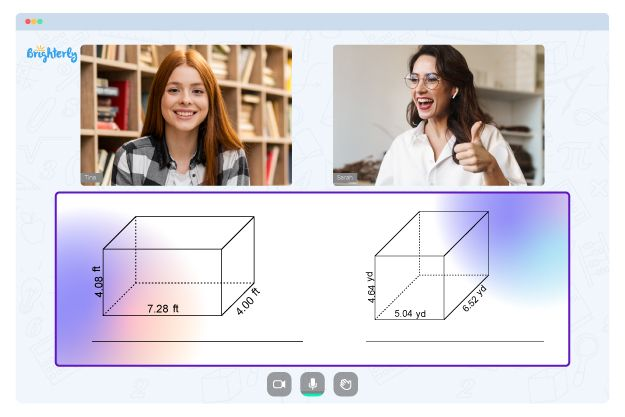# Properties Of Parallelograms Worksheets

When kids start learning geometry, they encounter different shapes, including parallelograms. A parallelogram is a quadrilateral with equal and parallel sides on opposite sides. The lengths and the angles of a parallelogram are equal. Kids need the properties of a parallelogram worksheet; it will help them scale through the complexities of understanding the shape.

## Benefits of parallelogram worksheets

Using properties of parallelogram worksheets to teach your kids has some benefits, and here they are:

### It helps the kids draw the parallelogram easily

When drawing a parallelogram, kids need clarification about what lines match and what angles should look like the other. With the worksheet and consistent practice, the kids can measure each side correctly to draw it and solve for the angles when needed.

### It helps them recognize the shape in real life

One of the ways to teach children about shapes is to show them the shapes in real life. With the properties of parallelograms worksheet, kids can master the look of an image enough to recognize it when they see it again.

Math for Kids

Is Your Child Struggling With Math?
1:1 Online Math Tutoring### It decomplexifies the idea behind parallelograms

At first glance, a parallelogram is a slanted rectangle, but kids learn as they progress in class that it is more than that. In tests and exams, they have to be accurate with measurements and diagrams. They can only do that if they have practiced using the properties of parallelograms worksheet answer key over time.

### It makes teaching easier for teachers and tutors

Teachers cannot spend the entire day introducing parallelograms to kids because they have to teach other things. Using properties of parallelogram worksheet answers ensures that the kids can practice independently, away from the teachers. This way, teachers can easily focus on whatever issue the children have with specific areas of understanding parallelograms instead of taking every class from scratch again.### Properties Of Parallelograms Worksheets PDF

Properties Of Parallelograms Worksheet### Properties Of Parallelograms Worksheets PDF### Properties Of Parallelograms Worksheets PDF

Properties Of A Parallelogram Worksheet### Properties Of Parallelograms Worksheets PDF

Properties Of Parallelograms Worksheet Answer Key

There are numerous properties of parallelogram worksheets in printable PDFs available for kids. When teaching kids in class, use properties of parallelograms worksheet answers PDF types to teach them. If you are setting homework for the kids using the properties of parallelograms worksheet answers, ensure that they try to solve the problems independently before using the solutions written on the back of the worksheet.

Troubles with Geometry?• Does your child need extra support with understanding geometry lessons?
• Start lessons with an online tutor.

Is your child finding it hard to master geometry? An online tutor could be of help.